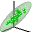#### File Exchange > Graphing >    3D Confidence EllipsoidAuthor:
OriginLab Technical Support
12/18/2015
Last Update:
5/31/2019
696
Total Ratings:
15
File Size:
66 KB
Average Rating:File Name:
Plot3dConf...id.opx
File Version:
2.00
Minimum Versions:
Free
Summary:

Plot 3D confidence ellipsoid for 3D scatter data (PRO Only).

Description:

Purpose
This tool can be used to:

• Create a 3D confidence ellipsoid plot for the selected XYZ scatter plot.
• Create a 3D confidence ellipsoid plot for each group level when the levels (3D scatter plots) are color indexed.
• Output principal axes and equations for 3D confidence ellipsoid.

Installation
Download the file Plot3dConfEllipsoid.opx, and then drag-and-drop onto the Origin workspace. An icon will appear in the Apps Gallery window.
NOTE: This tool requires OriginPro, and Show Principal Axes and Equations feature requires minimum version of Origin 2019b.

Operation

1. Click and select an XYZ scatter plot in a graph for which you want to create an ellipsoid. If no plot is selected, the tool will use the active plot in the graph window as input.
2. Click the 3D Confidence Ellipsoid icon in the Apps Gallery window to open the dialog.
3. In the opened dialog, set Confidence Level and Grid Size and click OK.
4. A transparent ellipsoid will be created in the graph window (Hint: To modify the Transparency setting, right-click on the ellipsoid plot in Object Manager and choose Plot Details) .
5. For Origin 2019b or higher versons, Show Principal Axes and Equations check box is available at the bottom of the dialog. Check the check box, click OK, three principal axes for each confidence ellipsoid will be added in the graph, and an HTML report will be created to show semi-axes and equations for these principal axes.

Algorithm
Assume $$\bar{X}$$ and S are the sample mean vector and covariance matrix for n samples with 3 variables, $$100(1-\alpha)\%$$ confidence ellipsoid can be calculated using Hotelling's T-squared ($$T^2$$) distribution:
$$(X-\bar{X})'S^{-1}(X-\bar{X})=\frac{3(n-1)}{(n-3)n}F_{\text{inv}}(1-\alpha,3,n-3)$$,
where $$F_{\text{inv}}$$ is the inverse F cumulative distribution function.
$$100(1-\alpha)\%$$ prediction ellipsoid can be given as:
$$(X-\bar{X})'S^{-1}(X-\bar{X})=\frac{3(n+1)(n-1)}{(n-3)n}F_{\text{inv}}(1-\alpha,3,n-3)$$.

NOTES:

• If the graph layer has more than one Z dataset plotted, the ellipsoid is added only to the selected XYZ scatter plot or the active plot if no plot is selected. You can add ellipsoids to other XYZ scatter plots in turn by clicking on the plot in the graph window or by selecting it in Object Manager, then relaunching the tool.
• If you have specified a grouping column to be used for index colors for the XYZ scatter plots (open Plot Details and click the Symbol tab, then select a group column in Color: By Points: Indexing.), the tool will create one confidence ellipsoid plot for each group level. Each ellipsoid will be color-matched to its scatter plot.

v2.0 5/28/2019 Updated to output principal axes and equations for the confidence ellipsoid.
v1.4 3/25/2019 Updated to support dependent group plot.
v1.3 8/16/2018 Updated to make it compatible with Origin 2019.
v1.2 Updated to make it compatible with Origin 2017.
v1.1 Fixed bug related to very large datasets.

04/12/2023OriginLabHi shipeng.jia,
For multiple scatter plots, you have to swtch the active plot to add the ellipsoids one by one.
Please see the first point in Note of this app webpage.
Thanks,
Shirley

04/12/2023shipeng.jiaI have more than one group but I can only plot one of them, why? I am using 202309/15/2022zyt12345606/30/2021JacquelineheHi yeukaikatanda,
Thanks!

06/29/2021yeukaikatandaThis new version doesn't work. There are no options to make the ellipsoid transparent or move it behind the scatter plot. Disappointed, please bring back the old version.01/09/2021gotodaiki

04/27/2020zhanghl11283D Confidence Ellipsoid11/21/2019Liqing Kou10/01/2019kang8How to select data within / outside of the ellipsoid?01/28/20191 2